American Journal of Theoretical and Applied Statistics
Volume 5, Issue 5, September 2016, Pages: 290-296

Estimating Survivor Function Using Adjusted Product Limit Estimator in the Presence of Ties

Job Isaac Mukangai*, Leo Odiwuor Odongo

Department of Statistics and Actuarial Science, Kenyatta University, Nairobi, Kenya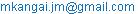(J. I. Mukangai)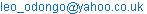(L. O. Odongo)

*Corresponding author

Job Isaac Mukangai, Leo Odiwuor Odongo. Estimating Survivor Function Using Adjusted Product Limit Estimator in the Presence of Ties. American Journal of Theoretical and Applied Statistics. Vol. 5, No. 5, 2016, pp. 290-296. doi: 10.11648/j.ajtas.20160505.17

Received: July 21, 2016; Accepted: August 1, 2016; Published: August 21, 2016

Abstract: We develop an adjusted Product Limit estimator for estimating survival probabilities in the presence of ties that incorporates censored individuals using the proportion of failing for uncensored individuals. We also develop a variance estimator of the adjusted Product Limit estimator for calculating confidence intervals. Simulation studies are carried out to assess the performance of the developed estimator in comparison to the performance of Kaplan-Meier and modified Kaplan-Meier estimators. Some simulation results are presented and one real data is used for illustration. The results indicate that the proposed estimator out performs the other estimators in estimating survival probabilities in presence of ties.

Keywords: Survival Analysis, Censored Data, Product Limit Estimator, Modified Kaplan-Meier

1. Introduction

Survival analysis is the phrase used to describe the analysis of data that correspond to time from a well-defined time origin until the occurrence of some particular event of interest or end point as in . Its techniques play increasing important roles in biostatistics, modern medical research, engineering, demography, among others. References to these applications may be found, among others, in [2, 3].

Kaplan and Meier  introduced Product Limit (PL) estimator, also known as Kaplan-Meier (KM) estimator, which has been in use since then as the standard estimator for estimating survival probabilities for censored data. The major limitation of PL estimator is that it ignores censored individuals incase ties between event and censoring times are observed. Because of this limitation, modified Kaplan-Meier (MKM) estimator discussed in  was suggested based on the arithmetic mean of the censored individuals and the survival probability for reduced sample size, considering this probability to be a single observation. The problem with MKM estimator is that the survival probabilities obtained are greater than survival probabilities obtained when censored individuals are ignored: this is unrealistic since some of censored individuals might fail leading to a decrease in survival probabilities and if none of the censored individuals fails then the survival probabilities ought to remain unchanged. Consequently, KM estimator overestimate survival probabilities [6-8] and this might be due to ignoring censored individuals in the presence of ties. Due to these drawbacks, in this article we propose an adjusted Product Limit estimator (APLE) that incorporates censored individuals in the presence of ties using the proportion of failing for uncensored individuals. The proposed estimator works consistently for all possible situations, that is, from light to heavy censoring and for small as well as large sample sizes. The rest of the paper is organized as follows: the proposed estimator is derived in section 2; simulation study is carried out in section 3 to evaluate the performance of the proposed estimator and to compare it with other estimators suggested in the literature. In section 4 the estimators are applied to real data and lastly in section 5 we conclude and give some recommendations.

2. Estimation

The idea of incorporating censored individuals when estimating survival probabilities in the presence of ties is to make full use of the information contained in these censored individuals because some of them might fail, though not observed, leading to a decrease in survival probabilities. Thus, ignoring them might lead to an overestimation of survival probabilities and this can be of severe consequences in some situations like duration for failure of a machine, relapse of a disease or occurrence of a strike, among others.

2.1. Kaplan-Meier and Modified Kaplan-Meier Survival Functions

Let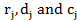be the number of individuals at risk, that fail, and that were censored at time tj respectively, then conventional Kaplan-Meier estimate of the survival function as in , is defined as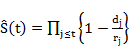(1)

While the MKM estimator in the presence of ties, as in , is given as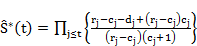(2)

In case of ties, both KM and MKM estimators overestimate the true survival probabilities. To overcome this, in the next subsection we develop an adjusted PL estimator.

Considering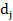and cj to occur together at time tj, we develop the APLE in the following steps: first, we ignore the censored units to get the estimated probability of failing for uncensored ones as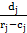.

In order to incorporate the censored units in presence of ties, we use this estimated probability of failing for fully observed units to estimate the expected number of units that fail out of the censored units at time tj; we have made the assumption that the two sets, censored and uncensored, are from same random sample, thus assumed to be positively correlated: the rate of failing in an unobserved set is likely to be similar to that in the observed one.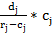Expected number of units that fails out of the censored ones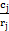Estimated probability of being censored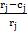Estimated probability of not being censored

Now, we get the estimated probability of a unit failing at time tj by summing the probabilities of it failing when it is censored or failing when it is not censored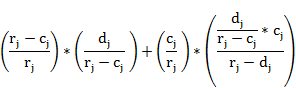Where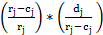represent the estimated probability of a unit not being censored and failing while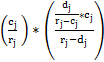is the estimated probability of it being censored and failing.

The expression simplifies to: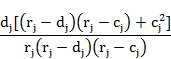To get the probability of surviving (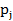) at time tj, we subtract the probability of failing at this time from one.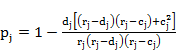(3)

Using the concept of Product Limit probability, we derive the Adjusted Product Limit survival function as follows: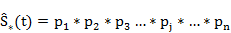(4)

It implies that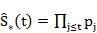(5)

Replacing (3) in (5), we get the Adjusted Product Limit Estimator as: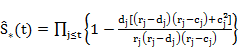(6)

2.3. Variance Estimator of Adjusted Product Limit Estimator

We derive the variance estimator for the proposed survival function using the delta method in . Considering Equation (5), taking log and variance both sides, we obtain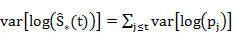(7)

Applying the delta method on the r.h.s of (7) we get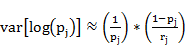(8)

From (7) and (8), it follows that: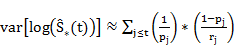(9)

A further application of delta method on the l.h.s of (7), we have: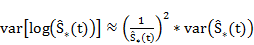(10)

Equating the r.h.s of (9) and (10) gives,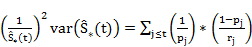(11)

On rearranging we get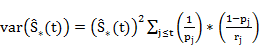(12)

Substituting forin (12) and simplifying, we get the variance of the Adjusted Product Limit Estimator as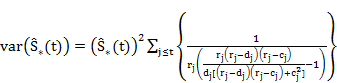(13)

The standard error of the Adjusted Product Limit estimate of the survival function is the square root of the estimated variance of the estimate.

In the absence of ties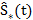=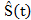, likewise, the estimated standard error for the adjusted Product Limit estimator equals to the standard error of the Greenwood’s formula in absence of ties.

3. Simulation Study

In this section we have carried out a simulation study to evaluate the performance of the proposed survivor function estimator and to compare it with other estimators suggested in the literature. Though time has been considered to be discrete in this study, we have used continuous survival distributions to draw survival and censoring times in R statistical package . Thereafter, we have converted the continuous times into discrete times by choosing one and/or zero decimal points: Both decimal points allow for inclusion of tie-case, thus, there is no difference of considering either one or zero.

We have simulated data of different sample size drawn from Weibull,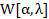and Log-logistic, Log-L[α, λ] survival distribution. Censoring, random censoring, was done using the uniform distribution ranging from 0 to b (for b=0.5, 0.8, 1.2, 1.5, 2 and 20). We have used different values of α, λ and b, different sample sizes with different percentages of censoring and simulated data using Weibull and Log-logistic distributions functions so as to assess the performance of the proposed estimator in different situations. Our simulated results are summarized in Tables 1-6, which gives the survival probabilities and standard errors for the estimators and also Survival curves for the three estimators are presented; since the results are similar, we have only presented some for illustration.

Tables 1 and 2 give estimated survival probabilities and standard errors for the three estimators for small samples with light censoring; for two different survival distributions considered in the two tables, it can be seen that both estimators give similar results: APLE estimates are the smallest while MKM estimates are the highest and estimates differs only as from the time a tie is observed.

Survival probability of zero may be preferred at the last observation time since no individual is expected to survive indefinite period of time. In Tables 1 and 5 estimates for all the three estimators go to zero since an event is observed at the last observation time, if last observation is censoring all the three estimators do not give survival probability, see Tables 3 and 6. But APLE estimates go to zero also when a tie is observed at the last observation time, see Tables 2 and 4, this shows that APLE is generally a better estimator than the other two estimators.

As was mentioned in section 1, MKM overestimate survival probabilities in the presence of ties: In Table 3 at time 0.0, 40 individuals were at risk, 2 failed and 1 was censored; using probability theorem, estimated probability of failing at this time is 2/40=0.05 (assuming that censored individual survived) and that of surviving is 1-0.05=0.95 (as obtained by KM estimator). Suppose the censored individual fail, then the estimated probability of failing is 3/40=0.075 and that of surviving is 1-0.075=0.925 which is a decrease. There is no justifiable reason(s) that will cause an increase in survival probability when censored individuals in the presence of ties are incorporated: this is so because censored individuals can either survive or fail though not observed. From this example, we see that MKM estimator overestimate survival probabilities in the presence of ties. On the other hand, one cannot simply ignore the censored individuals when estimating survival probabilities in the presence of ties yet they are also at risk and can either fail or survive just like the uncensored ones. It will be necessary to assume that both sets, censored and uncensored, are positively correlated. For instance, in Table 6 at observation time 7, 5 individuals failed while 4 were censored: ignoring these censored individuals, the way KM estimator does, is like assuming that all of them survived; an assumption which cannot hold all the time. It follows that KM estimator also to some extend overestimate survival probabilities in the presence of ties. Thus, KM is not an appropriate estimator in case ties between event and censoring times are present.

As discussed in , KM is unbiased estimator of the survivor function for large sample size and as in ; KM estimates approaches the true value for the population sampled as sample size tends to infinity. Comparing survival curves in Figures 1 to 6 for the three estimators MKM still give biased results even for large sample sizes; See for instance Figures 4 and 5 where the samples are large. It can be seen in Figure 6 that survival curves of KM and APLE tends to overlap an indication that also APLE estimates approaches the true value for the population sampled as sample size tends to infinity, from this we may say that APLE is unbiased and a consistent estimator just like KM estimator. Lastly, comparing standard errors for the three estimators, the standard errors for the proposed estimator and for the conventional KM estimator are in a close agreement while MKM estimator underestimate standard errors on the left and overestimate on the right, see for instance Table 3 in column 8, 9 and 10 for standard errors of APLE, KM and MKM estimators respectively.

4. Application to Real Data

We use Leukaemia data set given in  to demonstrate the application of the proposed estimator. The data set consists of weeks in maintenance of remission, ignoring the placebo controls, is as follows: 6, 6, 6, 6+, 7, 9+, 10, 10+, 11+, 13, 16, 17+, 19+, 20+, 22, 23, 25+, 32+, 32+, 34+, and 35+. Where (+) denotes a censored observation. The results of Leukaemia data are reported in Table 7 and in Figure 7. From the results it can be seen that the three estimators still give estimates similar to ones obtained in simulation study such that MKM estimates are highest while APLE estimates are the smallest. In Table 7 at week 6, 3 individuals failed and 1 was censored; estimated probability of failing at that time is 3/21=0.1429 and that of surviving is 1-0.1429=0.8571 which is equal to KM estimate at that time. Suppose the censored individual fail, though not observed, then estimated probability of failing will be 4/21=0.1905 and surviving will be 1-0.1905=0.8095 which is a decrease. Since we are not sure about the status of the censored individual, we can only estimate his survivorship and the overall estimated probability of surviving at that week must be within the interval [0.8095, 0.8571] as obtained by APLE. We respectively obtain 0.85, 0.8537 and 0.8534 using reduced sample (RS), Actuarial and Joint risk estimators; see  for details on these other estimators. From this example, it is clear that our proposed survivor function estimator works better than KM and MKM estimators in terms of estimating survival probabilities in the presence of ties.

In respect to standard errors, it can be seen in Table 7 that standard errors obtained using Greenwood variance estimator, discussed in , and that obtained using our proposed variance estimator are in a close agreement. In addition, if the results in Table 7 are rounded off, say to two decimal places, we notice that APLE and KM estimates are equal: It is justifiable to obtain such results because there are only two ties, at week 6 and 10, with one censored individual in each case, such a small number of ties and of the censored individual can’t cause much difference in the estimated survival probabilities. Therefore, MKM estimator overestimate survival probabilities in the presence of ties right from the start see also Figure 7 for details.

Table 1. Survival Probabilities and Standard Errors for APLE, KM and MKM for n=20 & 20% censoring; W [2, 1.5].

 tj rj dj cj APLE KM MKM se.APLE se.KM se.MKM 0.2 20 2 0 0.900000 0.900000 0.900000 0.067082 0.067082 0.067082 0.4 18 2 0 0.800000 0.800000 0.800000 0.089443 0.089443 0.089443 0.5 16 1 1 0.749778 0.750000 0.773333 0.096853 0.096825 0.093619 0.6 14 1 0 0.696222 0.696429 0.718095 0.103690 0.103675 0.101933 0.7 13 3 0 0.535556 0.535714 0.552381 0.113934 0.113942 0.114846 1.0 9 1 0 0.476049 0.476190 0.491005 0.115776 0.115791 0.117345 1.4 6 1 0 0.396708 0.396825 0.409171 0.120641 0.120664 0.123057 1.5 5 1 0 0.317366 0.317460 0.327337 0.119795 0.119822 0.122674 1.8 4 2 0 0.158683 0.158730 0.163668 0.099412 0.099439 0.102270 2.3 2 1 0 0.079342 0.079365 0.081834 0.074955 0.074976 0.077222 3.1 1 1 0 0.000000 0.000000 0.000000 N/A N/A N/A

NOTE: se.APLE, se.KM and se.MKM are standard error for APLE, KM and MKM respectively; N/A means not applicable.

Table 2. Survival Probabilities and Standard Errors for APLE, KM and MKM for n=10 & 20% censoring; Log L [1, 0.2].

 tj rj dj cj APLE KM MKM se.APLE se.KM se.MKM 0.7 10 2 0 0.80000 0.800000 0.800000 0.126491 0.126491 0.126491 0.8 8 1 0 0.70000 0.700000 0.700000 0.144914 0.144914 0.144914 0.9 7 1 0 0.60000 0.600000 0.600000 0.154919 0.154919 0.154919 1.0 6 1 0 0.50000 0.500000 0.500000 0.158114 0.158114 0.158114 1.1 5 1 1 0.39375 0.400000 0.437500 0.154503 0.154919 0.156874 1.2 3 1 0 0.26250 0.266667 0.291667 0.148640 0.150062 0.158479 1.3 2 1 1 0.00000 0.133333 0.145833 N/A 0.120493 0.130049

NOTE: se.APLE, se.KM and se.MKM are standard error for APLE, KM and MKM respectively; N/A means not applicable.

Table 3. Survival Probabilities and Standard Errors for APLE, KM and MKM for n=40 & 25% censoring; w [1.2, 0.5].

 tj rj dj cj APLE KM MKM se.APLE se.KM se.MKM 0.0 40 2 1 0.949966 0.950000 0.974359 0.034471 0.034460 0.024992 0.1 37 2 4 0.897905 0.898649 0.962549 0.048218 0.048060 0.030278 0.2 31 6 1 0.723886 0.724717 0.866294 0.074664 0.074622 0.058587 0.3 24 3 0 0.633400 0.634127 0.758007 0.081585 0.081590 0.077769 0.4 21 5 0 0.482590 0.483144 0.577529 0.085613 0.085662 0.092056 0.5 16 3 0 0.392105 0.392555 0.469242 0.084001 0.084064 0.093649 0.6 13 2 0 0.331781 0.332162 0.397051 0.081189 0.081257 0.092109 0.7 11 1 0 0.301619 0.301965 0.360956 0.079213 0.079283 0.090532 0.8 10 1 0 0.271457 0.271769 0.324860 0.076819 0.076890 0.088382 0.9 9 2 1 0.210056 0.211376 0.284253 0.070474 0.070674 0.085224 1.0 6 1 1 0.173646 0.176146 0.255827 0.066692 0.067104 0.084233 1.1 4 2 1 0.072353 0.088073 0.170552 0.051033 0.055362 0.082398

NOTE: se.APLE, se.KM and se.MKM are standard error for APLE, KM and MKM respectively.

Table 4. Survival Probabilities and Standard Errors for APLE, KM and MKM for n=30 & 40% censoring; W[ 1.5, 3].

 tj rj dj cj APLE KM MKM se.APLE se.KM se.MKM 0.0 30 3 0 0.900000 0.900000 0.900000 0.054772 0.054772 0.054772 0.1 27 5 2 0.732121 0.733333 0.840000 0.080854 0.080737 0.066933 0.3 17 6 2 0.467461 0.474510 0.728000 0.099713 0.099768 0.090339 0.4 9 1 2 0.411811 0.421786 0.693333 0.101305 0.101663 0.100365 0.5 6 1 1 0.340431 0.351489 0.624000 0.105182 0.106280 0.123975 0.6 4 1 0 0.255323 0.263617 0.468000 0.107961 0.110204 0.164005 0.7 3 1 2 0.000000 0.175744 0.312000 N/A 0.102691 0.167864

NOTE: se.APLE, se.KM and se.MKM are standard error for APLE, KM and MKM respectively; N/A means not applicable.

Table 5. Survival Probabilities and Standard Errors for APLE, KM and MKM for n=30 & 23.3% censoring; Log-L [1.2, 2].

 tj rj dj cj APLE KM MKM se.APLE se.KM se.MKM 0.4 27 1 1 0.962908 0.962963 0.980769 0.036371 0.036345 0.026430 0.5 25 1 0 0.924392 0.924444 0.941538 0.051413 0.051398 0.046057 0.7 24 4 0 0.770327 0.770370 0.784615 0.082345 0.082341 0.081261 0.8 20 1 1 0.731704 0.731852 0.763968 0.086780 0.086767 0.083959 0.9 18 1 0 0.691053 0.691193 0.721525 0.090983 0.090975 0.089380 1.0 17 6 1 0.445766 0.447243 0.586239 0.099379 0.099414 0.099695 1.1 10 4 0 0.267460 0.268346 0.351743 0.091238 0.091425 0.108749 1.2 6 1 1 0.221100 0.223621 0.316569 0.086006 0.086438 0.106935 1.3 4 2 0 0.110550 0.111811 0.158285 0.070033 0.070663 0.095511 1.4 2 1 0 0.055275 0.055905 0.079142 0.052477 0.053019 0.073568 1.5 1 1 0 0.000000 0.000000 0.000000 N/A N/A N/A

NOTE: se.APLE, se.KM and se.MKM are standard error for APLE, KM and MKM respectively; N/A means not applicable.

Table 6. Survival Probabilities and Standard Errors for APLE, KM and MKM for n=60 & 53.3% censoring; Log-L [10, 1.2].

 tj rj dj cj APLE KM MKM se.APLE se.KM se.MKM 4 60 1 0 0.983333 0.983333 0.983333 0.016527 0.016527 0.016527 7 48 5 4 0.880037 0.880903 0.960985 0.045963 0.045815 0.026614 8 39 1 3 0.857323 0.858316 0.954311 0.050043 0.049899 0.029356 9 35 3 7 0.779820 0.784746 0.941530 0.061634 0.061081 0.034390 10 25 11 0 0.436699 0.439458 0.527257 0.084764 0.085086 0.095436 11 14 4 2 0.307769 0.313898 0.468673 0.080019 0.080678 0.095696 12 8 2 2 0.222277 0.235424 0.416598 0.075599 0.077270 0.099737 13 4 1 2 0.129662 0.176568 0.347165 0.070334 0.077178 0.113728

NOTE: se.APLE, se.KM and se.MKM are standard error for APLE, KM and MKM respectively.

Table 7. Survival Probabilities and Standard Errors for APLE, KM and MKM for Leukaemia data set with n=21 & 57% censoring.

 tj rj dj cj APLE KM MKM se.APLE se.KM se.MKM 6 21 3 1 0.856746 0.857143 0.925000 0.076449 0.076360 0.057477 7 17 1 0 0.806349 0.806723 0.870588 0.086991 0.086935 0.075583 10 15 1 1 0.752318 0.752941 0.839496 0.096422 0.096350 0.083977 13 12 1 0 0.689625 0.690196 0.769538 0.106842 0.106815 0.102040 16 11 1 0 0.626932 0.627451 0.699580 0.114049 0.114054 0.114255 22 7 1 0 0.537370 0.537815 0.599640 0.128186 0.128234 0.134729 23 6 1 0 0.447809 0.448179 0.499700 0.134519 0.134591 0.144668

NOTE: se.APLE, se.KM and se.MKM are standard error for APLE, KM and MKM respectively.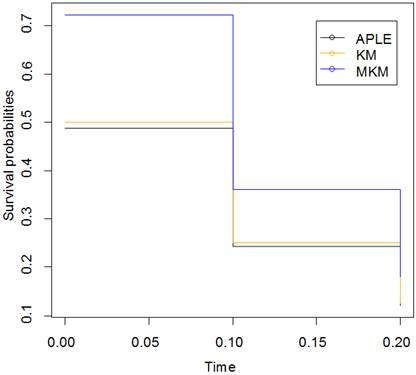Fig. 1. Survival curves for APLE, KM and MKM for n=10 & 20% censoring; W [0.5, 1.5].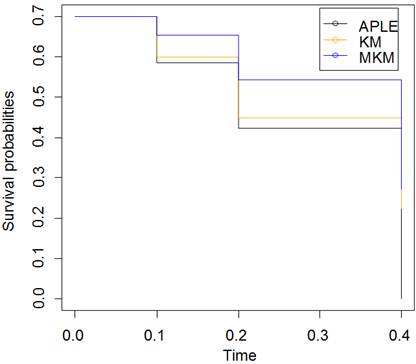Fig. 2. Survival curves for APLE, KM and MKM for n=10 & 40% censoring; W [0.5, 1.5].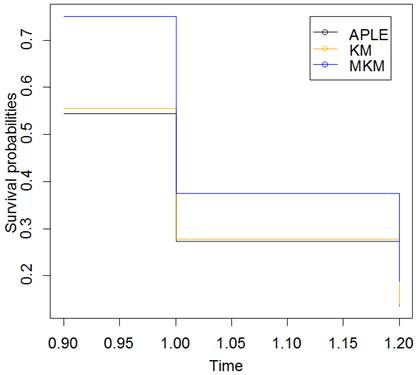Fig. 3. Survival curves for APLE, KM and MKM for n=15 & 53.3% censoring; Log-L [1, 0.2].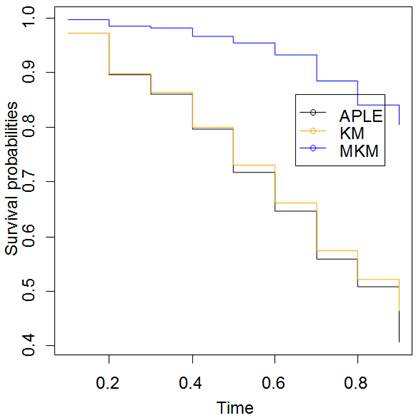Fig. 4. Survival curves for APLE, KM and MKM for n=80 & 74% censoring; W [0.5, 5].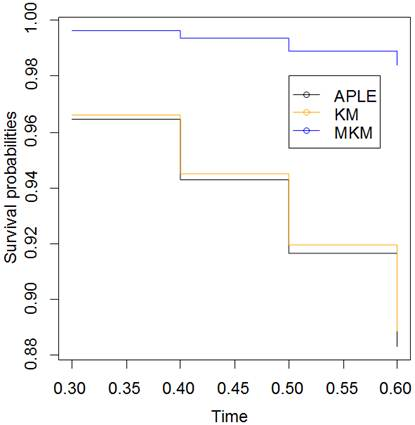Fig. 5. Survival curves for APLE, KM and MKM for n=80, 94% censoring; log-L [1, 0.2].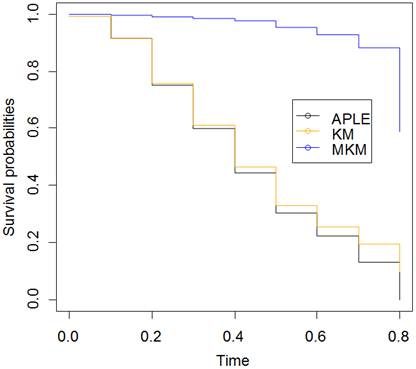Fig. 6. Survival curves for APLE, KM and MKM for n=400 & 51.5% censoring; W [2, 0.5].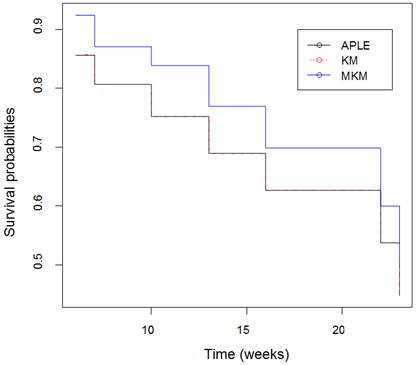Fig. 7. Survival curves for APLE, KM and MKM for Leukaemia data set with n=21 & 57% censoring.

5. Conclusion and Recommendation

This article considered the problem of incorporating censored individuals in calculating survival probabilities in the presence of ties. We developed an adjusted PL estimator and also a variance estimator for the developed estimator for calculating confidence intervals. The performance of the developed estimator, KM and MKM estimator were compared using both simulated and real data and it is observed that the performance of the proposed estimator is quite satisfactory. Our main conclusion is that MKM estimator overestimate survival probabilities while KM is not appropriate in case of ties between censoring and event times.

In calculating KM estimates in the presence of ties, censored individuals are ignored, and thus the information contained in the censored individuals is not utilized while in calculating APLE estimates, both censored and uncensored individuals are considered. So in this way, APLE estimates use all data and may be preferable to KM estimates. We therefore, recommend using the proposed estimator in calculating survival probabilities in the presence of ties.

Lastly, in this article ungrouped survival data drawn from Weibull and Log-logistic survival distributions were considered and observed that the results are similar. Extending the proposed estimator to grouped data and to data drawn from other survival distributions like lognormal, exponential, among others might be fruitful areas of future research.

References

1. Collett, D. (1994). Modeling Survival Data in Medical Research (1st edn.). London: Chapman & Hall/CRC.
2. Klein, J. P. and Goel, P. K. (2013). Survival Analysis: State of the art. Springer-science+Business media
3. John P. K., Hans C. V. H., Joseph G. I. and Thomas H. S. (2014). Handbook of Survival Analysis. London: Chapman & Hall/CRC.
4. Kaplan, E. L. and Meier, P. (1958). Nonparametric estimation from incomplete observations, Journal of the Amer. Statist. Assoc. 53, 457-481.
5. Zaman, Q., Atif, M., Iqbal, M., Pfeiffer, K. P. and Rafiq, M. (2014). Estimation of Survival Probabilities in the Presence of Ties. Short Title: Survival Probabilities in the Presence of Ties. Life Science Journal; 11(10s), 155-164.
6. Lacny S., Todd W., Fiona C., Dereck J. R., Peter D. F., William A. G. and Deborah A. M. (2015). Kaplan-Meier Survival Analysis Overestimates the Risk of Revision Arthroplasty. A Meta-analysis. Clin Orthop Relat Res; 473, 3431–3442.
7. Biau D. J., Latouche A., and Porcher R. (2007). Competing events influence estimated survival probability—when is Kaplan-Meier analysis appropriate? Clin Orthop Relat Res. 462, 229–233.
8. Pintilie M. (2006). Competing Risks: A Practical Perspective. West Sussex: John Wiley & Sons.
9. R Core Team (version 3.3.0). R: A language and environment for statistical computing. R Foundation for Statistical Computing, Vienna, Austria.URL http://www.R-project.org
10. Maller, R. and Zhou, X. (1996). Survival Analysis with Long-term survivors. John Wiley & Sons: Chichester
11. Freireich, E. J., Gehan, E., Schroeder, L. R., Wolman, I. J., Burgert, E. O., Mills, S. D., and Lee, S. (1963). The effect of 6-mercaptopurine on the duration of steroid-induced remissions in acute leukaemia: a model for evaluation of other potentially useful therapy. Blood; 21, 699-716.
12. Greenwood, M. (1926). The natural duration of cancer, Reports on public Health and Medical Subjects, His Majesty’s Stationery Office, London. 33, 18-26.

 Contents 1. 2. 2.1. 2.2. 2.3. 3. 4. 5.
Article ToolsAbstractPDF(803K)